# Electromotive force

Electromotive force, also called emf (denoted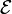$mathcal{E}$ and measured in volts), is the voltage developed by any source of electrical energy such as a battery or dynamo.

The word “force” in this case is not used to mean mechanical force, measured in newtons, but a potential, or energy per unit of charge, measured in volts.

In electromagnetic induction, emf can be defined around a closed loop as the electromagnetic work that would be transferred to a unit ofcharge if it travels once around that loop. (While the charge travels around the loop, it can simultaneously lose the energy via resistance into thermal energy.) For a time-varying magnetic flux impinging a loop, the electric potential scalar field is not defined due to circulating electric vector field, but nevertheless an emf does work that can be measured as a virtual electric potential around that loop.

In a two-terminal device (such as an electrochemical cell or electromagnetic generator), the emf can be measured as the open-circuit potential difference across the two terminals. The potential difference thus created drives current flow if an external circuit is attached to the source of emf. When current flows, however, the potential difference across the terminals is no longer equal to the emf, but will be smaller because of the voltage drop within the device due to its internal resistance.

Devices that can provide emf include electrochemical cells, thermoelectric devices, solar cells and photodiodes, electrical generators,transformers, and even Van de Graaff generators. In nature, emf is generated whenever magnetic field fluctuations occur through a surface. An example for this is the variation in the Earth’s magnetic field during a geomagnetic storm, acting on anything on the surface of the planet, like an extended electrical grid.

In the case of a battery, charge separation that gives rise to a voltage difference is accomplished by chemical reactions at the electrodes. Chemically, by separating positive and negative charges, an electric field can be produced, leading to an electric potential difference. A voltaic cell can be thought of as having a “charge pump” of atomic dimensions at each electrode, that is:

A source of emf can be thought of as a kind of charge pump that acts to move positive charge from a point of low potential through its interior to a point of high potential. … By chemical, mechanical or other means, the source of emf performs work dW on that charge to move it to the high potential terminal. The emf of the source is defined as the work dW done per charge dq: = dW/dq.

Around 1830 Faraday established that the reactions at each of the two electrode–electrolyte interfaces provide the “seat of emf” for the voltaic cell, that is, these reactions drive the current. In the open-circuit case, charge separation continues until the electrical field from the separated charges is sufficient to arrest the reactions. Years earlier, Volta, who had measured a contact potential difference at the metal-metal (electrode-electrode) interface of his cells, held the incorrect opinion that the fact of contact alone (without taking into account a chemical reaction) was the origin of the emf.

In the case of an electrical generator, a time-varying magnetic field inside the generator creates an electric field via electromagnetic induction, which in turn creates an energy difference between generator terminals. Charge separation takes place within the generator, with electrons flowing away from one terminal and toward the other, until, in the open-circuit case, sufficient electric field builds up to make further movement unfavorable. Again the emf is countered by the electrical voltage due to charge separation. If a load is attached, this voltage can drive a current. The general principle governing the emf in such electrical machines is Faraday’s law of induction.

## Notation and units of measurement

In a device without internal resistance, if an electric charge Q passes through that device, and gains an energy W, the net emf for that device is the energy gained per unitcharge, or W/Q. Like other measures of energy per charge, emf has SI units of volts, equivalent to joules per coulomb.

Electromotive force in electrostatic units is the statvolt (in the centimeter gram second system of units equal in amount to an erg per electrostatic unit of charge).

## Formal definitions of electromotive force

Inside a source of emf that is open-circuited, the conservative electrostatic field created by separation of charge exactly cancels the forces producing the emf. Thus, the emf has the same value but opposite sign as the integral of the electric field aligned with an internal path between two terminals A and B of a source of emf in open-circuit condition (the path is taken from the negative terminal to the positive terminal to yield a positive emf, indicating work done on the electrons moving in the circuit). Mathematically:$mathcal{E} = -int_{A}^{B} boldsymbol{E_{cs} cdot } d boldsymbol{ ell } ,$

where Ecs is the conservative electrostatic field created by the charge separation associated with the emf, d is an element of the path from terminal A to terminal B, and ‘·’ denotes the vector dot product. This equation applies only to locations A and B that are terminals, and does not apply to paths between points A and B with portions outside the source of emf. This equation involves the electrostatic electric field due to charge separation Ecs and does not involve (for example) any non-conservative component of electric field due to Faraday’s law of induction.

In the case of a closed path in the presence of a varying magnetic field, the integral of the electric field around a closed loop may be nonzero; one common application of the concept of emf, known as “induced emf” is the voltage induced in a such a loop. The “induced emf” around a stationary closed path C is:$mathcal{E}=oint_{C} boldsymbol{E cdot } d boldsymbol{ ell } ,$

where now E is the entire electric field, conservative and non-conservative, and the integral is around an arbitrary but stationary closed curve C through which there is a varying magnetic field. The electrostatic field does not contribute to the net emf around a circuit because the electrostatic portion of the electric field is conservative (that is, the work done against the field around a closed path is zero).

This definition can be extended to arbitrary sources of emf and moving paths C:$mathcal{E}=oint_{C}boldsymbol{ left[E + v times B right] cdot } d boldsymbol{ ell }$$+frac{1}{q}oint_{C}mathrm {mathbf{effective chemical forces cdot}} d boldsymbol{ ell }$$+frac{1}{q}oint_{C}mathrm {mathbf { effective thermal forces cdot}} d boldsymbol{ ell } ,$

which is a conceptual equation mainly, because the determination of the “effective forces” is difficult.

## Electromotive force in thermodynamics

When multiplied by an amount of charge dZ the emf ℰ yields a thermodynamic work term ℰdZ that is used in the formalism for the change in Gibbs free energy when charge is passed in a battery: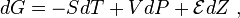$dG = -SdT + VdP + mathcal{E}dZ ,$

where G is the Gibb’s free energy, S is the entropy, V is the system volume, P is its pressure and T is its absolute temperature.

The combination ( ℰ, Z ) is an example of a conjugate pair of variables. At constant pressure the above relationship produces a Maxwell relation that links the change in open cell voltage with temperature T (a measurable quantity) to the change in entropy S when charge is passed isothermally and isobarically. The latter is closely related to the reaction entropy of the electrochemical reaction that lends the battery its power. This Maxwell relation is:$left(frac{partial mathcal{E}}{partial T}right)_Z= -left(frac{partial S}{partial Z}right)_T$

If a mole of ions goes into solution (for example, in a Daniell cell, as discussed below) the charge through the external circuit is: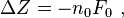$Delta Z = -n_0F_0 ,$

where n0 is the number of electrons/ion, and F0 is the Faraday constant and the minus sign indicates discharge of the cell. Assuming constant pressure and volume, the thermodynamic properties of the cell are related strictly to the behavior of its emf by:

where ΔH is the heat of reaction. The quantities on the right all are directly measurable.

## Electromotive force and voltage difference

An electrical voltage difference is sometimes called an emf. The points below illustrate the more formal usage, in terms of the distinction between emf and the voltage it generates:

1. For a circuit as a whole, such as one containing a resistor in series with a voltaic cell, electrical voltage does not contribute to the overall emf, because the voltage difference on going around a circuit is zero. (The ohmic IR drop plus the applied electrical voltage is zero. See Kirchhoff’s Law). The emf is due solely to the chemistry in the battery that causes charge separation, which in turn creates an electrical voltage that drives the current.
2. For a circuit consisting of an electrical generator that drives current through a resistor, the emf is due solely to a time-varying magnetic field that generates an electrical voltage that in turn drives the current. (The ohmic IR drop plus the applied electrical voltage again is zero. See Kirchhoff’s Law)
3. A transformer coupling two circuits may be considered a source of emf for one of the circuits, just as if it were caused by an electrical generator; this example illustrates the origin of the term “transformer emf”.
4. A photodiode or solar cell may be considered as a source of emf, similar to a battery, resulting in an electrical voltage generated by charge separation driven by light rather than chemical reaction.
5. Other devices that produce emf are fuel cells, thermocouples, and thermopiles.

In the case of an open circuit, the electric charge that has been separated by the mechanism generating the emf creates an electric field opposing the separation mechanism. For example, the chemical reaction in a voltaic cell stops when the opposing electric field at each electrode is strong enough to arrest the reactions. A larger opposing field can reverse the reactions in what are called reversible cells.

The electric charge that has been separated creates an electric potential difference that can be measured with a voltmeter between the terminals of the device. The magnitude of the emf for the battery (or other source) is the value of this ‘open circuit’ voltage. When the battery is charging or discharging, the emf itself cannot be measured directly using the external voltage because some voltage is lost inside the source. It can, however, be inferred from a measurement of the current I and voltage difference V, provided that the internal resistance r already has been measured:  = V + Ir.

## Electromotive force generation

### Chemical sourcesA typical reaction path requires the initial reactants to cross an energy barrier, enter an intermediate state and finally emerge in a lower energy configuration. If charge separation is involved, this energy difference can result in an emf. See Bergmann et al. and Transition state.Galvanic cell using a salt bridge

The question of how batteries (galvanic cells) generate an emf is one that occupied scientists for most of the 19th century. The “seat of the electromotive force” was eventually determined by Walther Nernst to be primarily at the interfaces between the electrodes and the electrolyte.

Molecules are groups of atoms held together by chemical bonds, and these bonds consist of electrical forces between electrons (negative) and protons (positive). The molecule in isolation is a stable entity, but when different molecules are brought together, some types of molecules are able to steal electrons from others, resulting in charge separation. This redistribution of charge is accompanied by a change in energy of the system, and a reconfiguration of the atoms in the molecules. The gain of an electron is termed “reduction” and the loss of an electron is termed “oxidation”. Reactions in which such electron exchange occurs (which are the basis for batteries) are called reduction-oxidation reactions or redox reactions. In a battery, one electrode is composed of material that gains electrons from the solute, and the other electrode loses electrons, because of these fundamental molecular attributes. The same behavior can be seen in atoms themselves, and their ability to steal electrons is referred to as their electronegativity.

As an example, a Daniell cell consists of a zinc anode (an electron collector), which dissolves into a zinc sulfate solution, the dissolving zinc leaving behind its electrons in the electrode according to the oxidation reaction (s = solid electrode; aq = aqueous solution):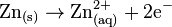$mathrm{Zn_{(s)} rightarrow Zn^{2+}_{(aq)} + 2 e ^- }$

The zinc sulfate is an electrolyte, that is, a solution in which the components consist of ions, in this case zinc ions$mathrm{Zn}_{} ^{2+}$, and sulfate ions$mathrm{SO}_4^{2-}$.

At the cathode, the copper ions in a copper sulfate electrolyte adopt electrons from the electrode by the reduction reaction:$mathrm{Cu^{2+}_{(aq)} + 2 e^- rightarrow Cu_{(s)} }$

and the thus-neutralized copper plates onto the electrode. (A detailed discussion of the microscopic process of electron transfer between an electrode and the ions in an electrolyte may be found in Conway.)

The electrons pass through the external circuit (light bulb in figure), while the ions pass through the salt bridge to maintain charge balance. In the process the zinc anode is dissolved while the copper electrode is plated with copper. If the light bulb is removed (open circuit) the emf between the electrodes is opposed by the electric field due to charge separation, and the reactions stop.

At 273 K, the emf = 1.0934 V, with a temperature coefficient of d/dT = −4.53×10−4 V/K.

#### Voltaic cells

Volta developed the voltaic cell about 1792, and presented his work March 20, 1800. Volta correctly identified the role of dissimilar electrodes in producing the voltage, but incorrectly dismissed any role for the electrolyte. Volta ordered the metals in a ‘tension series’, “that is to say in an order such that any one in the list becomes positive when in contact with any one that succeeds, but negative by contact with any one that precedes it.” A typical symbolic convention in a schematic of this circuit ( –||– ) would have a long electrode 1 and a short electrode 2, to indicate that electrode 1 dominates. Volta’s law about opposing electrode emfs means that, given ten electrodes (for example, zinc and nine other materials), which can be used to produce 45 types of voltaic cells (10 × 9/2), only nine relative measurements (for example, copper and each of the nine others) are needed to get all 45 possible emfs that these ten electrodes can produce.

#### Electromotive force of cells

The electromotive force produced by primary (single-use) and secondary (rechargeable) cells is usually of the order of a few volts. The figures quoted below are nominal, because emf varies according to the size of the load and the state of exhaustion of the cell.

Emf Cell chemistry
1.2 V nickel–metal hydride
1.5 V zinc–carbon
3.6 V to 3.7 V lithium-ion

### Electromagnetic induction

The principle of electromagnetic induction, noted above, states that a time-dependent magnetic field produces a circulating electric field. A time-dependent magnetic field can be produced either by motion of a magnet relative to a circuit, by motion of a circuit relative to another circuit (at least one of these must be carrying a current), or by changing the current in a fixed circuit. The effect on the circuit itself, of changing the current, is known as self-induction; the effect on another circuit is known as mutual induction.

For a given circuit, the electromagnetically induced emf is determined purely by the rate of change of the magnetic flux through the circuit according to Faraday’s law of induction.

An emf is induced in a coil or conductor whenever there is change in the flux linkages. Depending on the way in which the changes are brought about, there are two types: When the conductor is moved in a stationary magnetic field to procure a change in the flux linkage, the emf is statically induced. The electromotive force generated by motion is often referred to as motional emf. When the change in flux linkage arises from a change in the magnetic field around the stationary conductor, the emf is dynamically induced.The electromotive force generated by a time-varying magnetic field is often referred to as transformer emf.

### Contact potentials

When two different solids are in contact, it is common that thermodynamic equilibrium requires one of the solids assume a higher electrical potential than the other, the contact potential. For example, dissimilar metals in contact produce what is known also as a contact electromotive force or Galvani potential. The magnitude of this potential difference often is expressed as a difference in Fermi levels in the two solids at charge neutrality, where the Fermi level (a name for the chemical potential of an electron system) describes the energy necessary to remove an electron from the body to some common point (such as ground). Evidently, if there is an energy advantage in taking an electron from one body to the other, then such a transfer will occur. The transfer causes a charge separation, with one body gaining electrons and the other losing electrons. This charge transfer causes a potential difference between the bodies, which partly cancels the potential coming from the contact, and therefore, charge transfer becomes more difficult as the charge separation increases. At thermodynamic equilibrium, the Fermi levels are equal (the electron removal energy is identical) and there is now a built-in electrostatic potential between the bodies. The original difference in Fermi levels, before contact, is referred to as the emf. The contact potential cannot drive steady current through a load attached to its terminals because that current would involve a charge transfer. No mechanism exists to continue such transfer and, hence, maintain a current, once equilibrium is attained.

One might inquire why the contact potential does not appear in Kirchhoff’s law of voltages as one contribution to the sum of potential drops. The customary answer is that any circuit involves not only a particular diode or junction, but also all the contact potentials due to wiring and so forth around the entire circuit. The sum of all the contact potentials is zero, and so they may be ignored in Kirchhoff’s law.

### Solar cellThe equivalent circuit of a solar cell; parasitic resistances are ignored in the discussion of the text.Solar cell voltage as a function of solar cell current delivered to a load for two light-induced currents IL; currents as a ratio with reverse saturation current I0. Compare with Fig. 1.4 in Nelson.

Operation of a solar cell can be understood from the equivalent circuit at right. Light, if it includes photons of sufficient energy (greater than the bandgap of the material), creates mobile electron–hole pairs in a semiconductor. Charge separation occurs because of a pre-existing electric field associated with the p-n junction in thermal equilibrium (a contact potential creates the field). This charge separation between positive holes and negative electrons across a p-n junction (a diode), yields a forward voltage, thephoto voltage, between the illuminated diode terminals. As has been noted earlier in the terminology section, the photo voltageis sometimes referred to as the photo emf, rather than distinguishing between the effect and the cause.

The light-induced charge separation creates a reverse current through the cell’s junction (that is, not in the direction that a diode normally conducts current), and the charge separation causes a photo voltage that drives current through any attached load. However, a side effect of this voltage is that it tends to forward bias the junction. At high enough levels, this forward bias of the junction will cause a forward current in the diode that subtracts from the current created by the light. Consequently, the greatest current is obtained under short-circuit conditions, and is denoted as IL (for light-induced current) in the equivalent circuit. Approximately this same current is obtained for forward voltages up to the point where the diode conduction becomes significant.

With this notation, the current-voltage relation for the illuminated diode is:$I = I_L -I_0 left( e^{qV/(mkT)} - 1 right) ,$

where I is the current delivered to the load, I0 is the reverse saturation current, and m the ideality factor, two parameters that depend on the solar cell construction and to some degree upon the voltage itself, and where kT/q is the thermal voltage (about 0.026 V at room temperature). This relation is plotted in the figure using a fixed value m = 2. Under open-circuit conditions (that is, as I → 0), the open-circuit voltage is the voltage at which forward bias of the junction is enough that the forward current completely balances the photocurrent. Rearrangement of the I–V equation provides the open-circuit voltage as: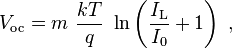$V_text{oc} = m frac{kT}{q} ln left( frac{I_text{L}}{I_0}+1 right) ,$

which is useful in indicating a logarithmic dependence of Voc upon the light-induced current. Typically, the open-circuit voltage is not more than about 0.5 V.

The value of the photo voltage when driving a load is variable. As shown in the figure, for a load resistance RL, the cell develops a voltage between the short-circuit value V = 0, I = IL and the open-circuit value Voc, I = 0, a value given by Ohm’s law V = I RL, where the current I is the difference between the short-circuit current and current due to forward bias of the junction, as indicated by the equivalent circuit (neglecting the parasitic resistances).

In contrast to the battery, at current levels near IL, the solar cell acts more like a current source rather than a voltage source. The current drawn is nearly fixed over a range of load voltages, at one electron per converted photon. The quantum efficiency, or probability of getting an electron of photocurrent per incident photon, depends not only upon the solar cell itself, but upon the spectrum of the light.

The diode possesses a “built-in potential” due to the contact potential difference between the two different materials on either side of the junction. This built-in potential is established when the junction is formed as a by-product of thermodynamic equilibrium. Once established, this potential difference cannot drive a current, however, as connecting a load does not upset this equilibrium. In contrast, the accumulation of excess electrons in one region and of excess holes in another due to illumination results in a photo voltage that does drive a current when a load is attached to the illuminated diode. As noted above, this photo voltage also forward biases the junction, and so reducesthe pre-existing field in the depletion region.

Source: Wikipedia and RCAS library